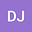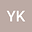Dynamics of an infinite age-structured particle system
••• Dominika Jasinska,
• Yuri Kozitsky
Dominika Jasinska
Maria Curie-Sklodowska University
Author ProfileYuri Kozitsky
Maria Curie-Sklodowska University
Author Profile## Abstract

The Markov evolution is studied of an infinite age-structured population of migrants arriving in and departing from a continuous habitat $X \subseteq\mathds{R}^d$ – at random and independently of each other. Each population member is characterized by its age $a\geq 0$ (time of presence in the population) and location $x\in X$. The population states are probability measures on the space of the corresponding marked configurations. The result of the paper is constructing the evolution $\mu_0 \to \mu_t$ of such states by solving a standard Fokker-Planck equation for this models. We also found a stationary state $\mu$ existing if the emigration rate is separated away from zero. It is then shown that $\mu_t$ weakly converges to $\mu$ as $t\to +\infty$.

#### Peer review status:ACCEPTED

18 Jan 2020Submitted to Mathematical Methods in the Applied Sciences
11 Aug 2020Submission Checks Completed
11 Aug 2020Assigned to Editor
24 Aug 2020Reviewer(s) Assigned
06 Dec 2020Review(s) Completed, Editorial Evaluation Pending
11 Dec 2020Editorial Decision: Revise Minor